# pzoch_vilnius2022.pdf

20. Sep 2022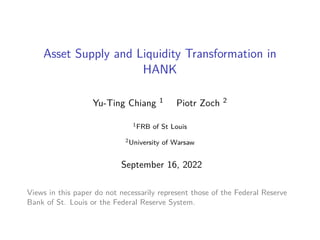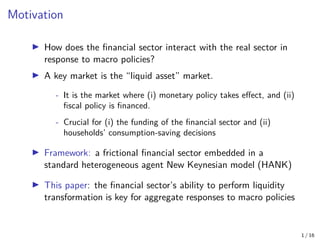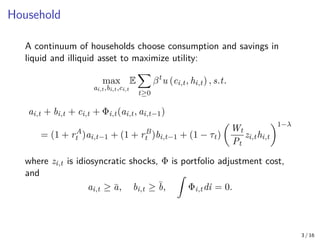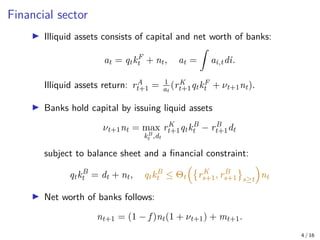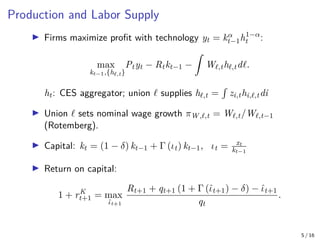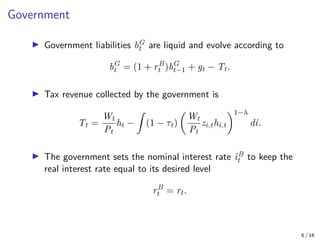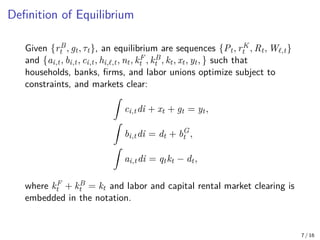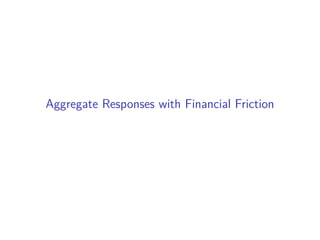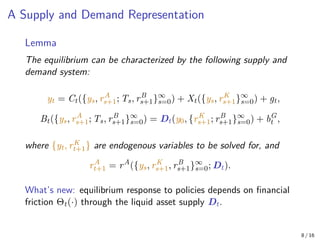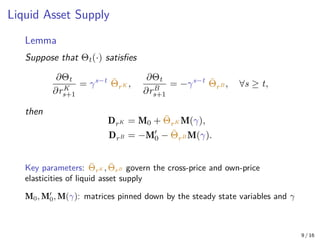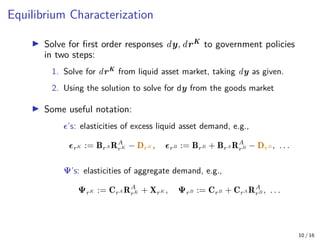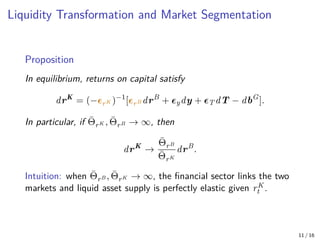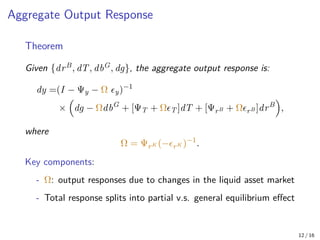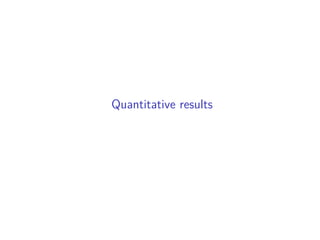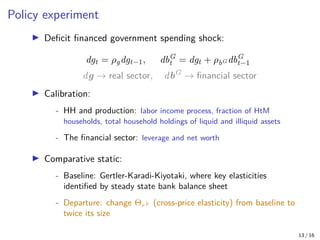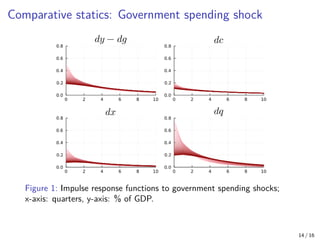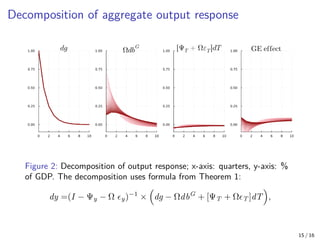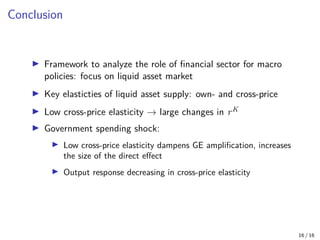1 von 20

### pzoch_vilnius2022.pdf

• 1. Asset Supply and Liquidity Transformation in HANK Yu-Ting Chiang 1 Piotr Zoch 2 1FRB of St Louis 2University of Warsaw September 16, 2022 Views in this paper do not necessarily represent those of the Federal Reserve Bank of St. Louis or the Federal Reserve System.
• 2. Motivation I How does the financial sector interact with the real sector in response to macro policies? I A key market is the “liquid asset” market. - It is the market where (i) monetary policy takes effect, and (ii) fiscal policy is financed. - Crucial for (i) the funding of the financial sector and (ii) households’ consumption-saving decisions I Framework: a frictional financial sector embedded in a standard heterogeneous agent New Keynesian model (HANK) I This paper: the financial sector’s ability to perform liquidity transformation is key for aggregate responses to macro policies 1 / 16
• 3. Result Main result: The role of financial sector in a large class of models summarized by two key elasticities of the liquid asset supply: Own-price: how much return on liquid assets (monetary policy) affects the real sector Cross-price: how much shocks to the real sector affect the financial sector Quantitative implications (deficit financed gov. spending): Small cross-price elasticity → large adjustment in return on capital: dampens GE amplification and increases direct effect of debt. 2 / 16
• 4. Model
• 5. Household A continuum of households choose consumption and savings in liquid and illiquid asset to maximize utility: max ai,t,bi,t,ci,t E X t≥0 βt u (ci,t, hi,t) , s.t. ai,t + bi,t + ci,t + Φi,t(ai,t, ai,t−1) = (1 + rA t )ai,t−1 + (1 + rB t )bi,t−1 + (1 − τt) Wt Pt zi,thi,t 1−λ where zi,t is idiosyncratic shocks, Φ is portfolio adjustment cost, and ai,t ≥ ā, bi,t ≥ b̄, Z Φi,tdi = 0. 3 / 16
• 6. Financial sector I Illiquid assets consists of capital and net worth of banks: at = qtkF t + nt, at = Z ai,tdi. Illiquid assets return: rA t+1 = 1 at (rK t+1qtkF t + νt+1nt). I Banks hold capital by issuing liquid assets νt+1nt = max kB t ,dt rK t+1qtkB t − rB t+1dt subject to balance sheet and a financial constraint: qtkB t = dt + nt, qtkB t ≤ Θt rK s+1, rB s+1 s≥t nt I Net worth of banks follows: nt+1 = (1 − f )nt(1 + νt+1) + mt+1. 4 / 16
• 7. Production and Labor Supply I Firms maximize profit with technology yt = kα t−1h1−α t : max kt−1,{h,t} Ptyt − Rtkt−1 − Z W,th,td. ht: CES aggregator; union  supplies h,t = R zi,thi,,tdi I Union  sets nominal wage growth πW,,t = W,t/W,t−1 (Rotemberg). I Capital: kt = (1 − δ) kt−1 + Γ (ιt) kt−1, ιt = xt kt−1 I Return on capital: 1 + rK t+1 = max ι̂t+1 Rt+1 + qt+1 (1 + Γ (ι̂t+1) − δ) − ι̂t+1 qt . 5 / 16
• 8. Government I Government liabilities bG t are liquid and evolve according to bG t = (1 + rB t )bG t−1 + gt − Tt. I Tax revenue collected by the government is Tt = Wt Pt ht − Z (1 − τt) Wt Pt zi,thi,t 1−λ di. I The government sets the nominal interest rate iB t to keep the real interest rate equal to its desired level rB t = rt. 6 / 16
• 9. Definition of Equilibrium Given {rB t , gt, τt}, an equilibrium are sequences {Pt, rK t , Rt, W,t} and {ai,t, bi,t, ci,t, hi,`,t, nt, kF t , kB t , kt, xt, yt, } such that households, banks, firms, and labor unions optimize subject to constraints, and markets clear: Z ci,tdi + xt + gt = yt, Z bi,tdi = dt + bG t , Z ai,tdi = qtkt − dt, where kF t + kB t = kt and labor and capital rental market clearing is embedded in the notation. 7 / 16
• 10. Aggregate Responses with Financial Friction
• 11. A Supply and Demand Representation Lemma The equilibrium can be characterized by the following supply and demand system: yt = Ct({ys, rA s+1; Ts, rB s+1}∞ s=0) + Xt({ys, rK s+1}∞ s=0) + gt, Bt({ys, rA s+1; Ts, rB s+1}∞ s=0) = Dt(y0, {rK s+1; rB s+1}∞ s=0) + bG t , where {yt, rK t+1} are endogenous variables to be solved for, and rA t+1 = rA ({ys, rK s+1, rB s+1}∞ s=0; Dt). What’s new: equilibrium response to policies depends on financial friction Θt(·) through the liquid asset supply Dt. 8 / 16
• 12. Liquid Asset Supply Lemma Suppose that Θt(·) satisfies ∂Θt ∂rK s+1 = γs−t Θ̄rK , ∂Θt ∂rB s+1 = −γs−t Θ̄rB , ∀s ≥ t, then DrK = M0 + Θ̄rK M(γ), DrB = −M0 0 − Θ̄rB M(γ). Key parameters: Θ̄rK , Θ̄rB govern the cross-price and own-price elasticities of liquid asset supply M0, M0 0, M(γ): matrices pinned down by the steady state variables and γ 9 / 16
• 13. Equilibrium Characterization I Solve for first order responses dy, drK to government policies in two steps: 1. Solve for drK from liquid asset market, taking dy as given. 2. Using the solution to solve for dy from the goods market I Some useful notation: ’s: elasticities of excess liquid asset demand, e.g., rK := BrA RA rK − DrK , rB := BrB + BrA RA rB − DrB , . . . Ψ’s: elasticities of aggregate demand, e.g., ΨrK := CrA RA rK + XrK , ΨrB := CrB + CrA RA rB , . . . 10 / 16
• 14. Liquidity Transformation and Market Segmentation Proposition In equilibrium, returns on capital satisfy drK = (−rK )−1 [rB drB + ydy + T dT − dbG ]. In particular, if Θ̄rK , Θ̄rB → ∞, then drK → Θ̄rB Θ̄rK drB . Intuition: when Θ̄rB , Θ̄rK → ∞, the financial sector links the two markets and liquid asset supply is perfectly elastic given rK t . 11 / 16
• 15. Aggregate Output Response Theorem Given {drB, dT, dbG, dg}, the aggregate output response is: dy =(I − Ψy − Ω y)−1 × dg − ΩdbG + [ΨT + ΩT ]dT + [ΨrB + ΩrB ]drB , where Ω = ΨrK (−rK )−1 . Key components: - Ω: output responses due to changes in the liquid asset market - Total response splits into partial v.s. general equilibrium effect 12 / 16
• 16. Quantitative results
• 17. Policy experiment I Deficit financed government spending shock: dgt = ρgdgt−1, dbG t = dgt + ρbG dbG t−1 dg → real sector, dbG → financial sector I Calibration: - HH and production: labor income process, fraction of HtM households, total household holdings of liquid and illiquid assets - The financial sector: leverage and net worth I Comparative static: - Baseline: Gertler-Karadi-Kiyotaki, where key elasticities identified by steady state bank balance sheet - Departure: change ΘrK (cross-price elasticity) from baseline to twice its size 13 / 16
• 18. Comparative statics: Government spending shock Figure 1: Impulse response functions to government spending shocks; x-axis: quarters, y-axis: % of GDP. 14 / 16
• 19. Decomposition of aggregate output response Figure 2: Decomposition of output response; x-axis: quarters, y-axis: % of GDP. The decomposition uses formula from Theorem 1: dy =(I − Ψy − Ω y)−1 × dg − ΩdbG + [ΨT + ΩT ]dT , 15 / 16
• 20. Conclusion I Framework to analyze the role of financial sector for macro policies: focus on liquid asset market I Key elasticties of liquid asset supply: own- and cross-price I Low cross-price elasticity → large changes in rK I Government spending shock: I Low cross-price elasticity dampens GE amplification, increases the size of the direct effect I Output response decreasing in cross-price elasticity 16 / 16